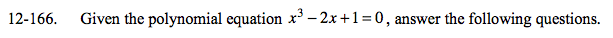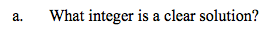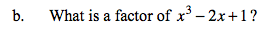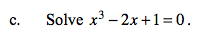### Home > A2C > Chapter 12 > Lesson 12.4.1 > Problem12-166

12-166.x = 1What is the factor for x = 1?If one factor is (x − 1), what is the second factor? Divide x3 − 2x + 1 by x − 1.

$x=1 \text{ or }x=\frac{-1\pm\sqrt{5}}{2}$